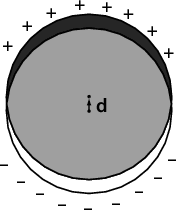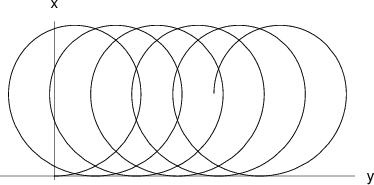17th of September, 2008

1. The potential must be the same everywhere inside the sphere. This condition is satisfied if the charge density is constant on the surface of the sphere and $0$ everywhere else. It can easily be seen that this configuration is a valid solution because bound charges can be induced only on the surface of a homogeneous and linear dielectric if the normal component of the electric field is non-zero there.

It can be proven that this is the only solution using an argument similar to that found in Griffiths’ book (page 118, second uniqueness theorem), but using the displacement vector $\stackrel{\to }{D}$ instead of $\stackrel{\to }{E}$.

If the sphere is pushed “deeper” into the dielectric (fig. 2a), the potential cannot stay unchanged because the electric field will no longer be parallel to the surface of the dielectric and bound charges will be induced. In the case illustrated on fig. 2b, the potential will not change.

If the total charge of the sphere is constant, the charges will redistribute on the surface of the sphere in such a way that the electric field can stay spherically symmetric. On the lower hemisphere the bound charges will reduce the total (bound + free) surface charge density by a factor of $\epsilon$. Thus, if the total free charge is , and the total charge is $Q$, . The potential will be reduced by a factor of $2∕\left(1+\epsilon \right)$ everywhere in space.

2. See Griffiths, page 194.
3. Carving a cavity inside a polarized dielectric is equivalent to superposing a region of equal but opposite polarization. Since the dielectric is uniformly polarized, all we need to do is find the electric field inside a uniformly polarized spherical, needle- and wafer-shaped region, and superpose this field with ${\stackrel{\to }{E}}_{0}$.

The polarization of the dielectric is ${\stackrel{\to }{P}}_{0}={\epsilon }_{0}\chi {\stackrel{\to }{E}}_{0}$ where $\chi ={\epsilon }_{r}-1$ is the susceptibility of the material. We shall need to consider regions with uniform polarization $-{\stackrel{\to }{P}}_{0}$.

1. Spherical region

What is the electric field inside a uniformly polarized sphere, of polarization $\stackrel{\to }{P}$?A uniformly polarized sphere can be imagined as two superposed uniformly but oppositely charged spheres, slightly displaced by $\stackrel{\to }{d}$. The electric field inside a uniformly charged sphere with charge density $\rho$, at position $\stackrel{\to }{r}$ relative to the sphere’s centre is

$\stackrel{\to }{E}\left(\stackrel{\to }{r}\right)=\frac{1}{{\epsilon }_{0}}\frac{\rho \stackrel{\to }{r}}{3}.$

The electric field inside the superposed spheres is

$\stackrel{\to }{E}\left(\stackrel{\to }{r}\right)=\frac{1}{{\epsilon }_{0}}\frac{\rho \left(\stackrel{\to }{d}+\stackrel{\to }{r}\right)}{3}-\frac{1}{{\epsilon }_{0}}\frac{\rho \stackrel{\to }{r}}{3}=\frac{1}{{\epsilon }_{0}}\frac{\rho \stackrel{\to }{d}}{3}.$

The polarization vector can be thought of as a density of electric dipole moments, so $\stackrel{\to }{P}=\rho \stackrel{\to }{d}$, and the electric field inside the polarized region is

$\stackrel{\to }{E}=\frac{1}{{\epsilon }_{0}}\frac{\stackrel{\to }{P}}{3}.$

The electric field in a spherical cavity is

$\stackrel{\to }{E}={\stackrel{\to }{E}}_{0}+\frac{1}{{\epsilon }_{0}}\frac{{\epsilon }_{0}\chi {\stackrel{\to }{E}}_{0}}{3}=\frac{2+{\epsilon }_{r}}{3}{\stackrel{\to }{E}}_{0}.$

2. Needle-shaped region Let us denote the tiny base surface of the needle with $A$. Then the bound charges induced by the polarization will be two point like charges of magnitude $AP$ at the tips of the needle.

Thus the electric field in the middle of a needle of length $L$ is

$E=2×\frac{1}{4\pi {\epsilon }_{0}}\frac{AP}{{\left(L∕2\right)}^{2}}.$

The electric field in a cavity is

$E={E}_{0}+\frac{2}{\pi {\epsilon }_{0}}\frac{A{\epsilon }_{0}\chi {E}_{0}}{{L}^{2}}={E}_{0}\left(1+\frac{2\chi }{\pi }\frac{A}{{L}^{2}}\right).$

3. Wafer-shaped region A polarized flat region is just like the capacitor: two parallel sheets of bound charges, with surface charge density $\sigma =P$. The electric field inside this region is just $E=\sigma ∕{\epsilon }_{0}=P∕{\epsilon }_{0}$.

Thus the electric field in a thin wafer shaped cavity is

$E={E}_{0}+{\epsilon }_{0}\chi {E}_{0}∕{\epsilon }_{0}={E}_{0}\left(1+\chi \right)={E}_{0}{\epsilon }_{r}.$

4. What is the dipole moment of a dielectric sphere placed in a homogeneous electric field ${\stackrel{\to }{E}}_{0}$? As shown in the previous exercise, the electric field created by a polarized sphere is . The total electric field in the sphere is and $\stackrel{\to }{P}={\epsilon }_{0}\chi \stackrel{\to }{E}={\epsilon }_{0}\left({\epsilon }_{r}-1\right)\stackrel{\to }{E}$, so

The dipole moment of a sphere of volume $V$ and polarization $\stackrel{\to }{P}$ is $\stackrel{\to }{p}=V\stackrel{\to }{P}$, so the polarizability of a sphere is $\alpha =3V{\epsilon }_{0}\frac{{\epsilon }_{r}-1}{{\epsilon }_{r}+2}$.

Let us assume that that all the droplets have volume $V$ and there are $N$ droplets per unit volume: $NV=\beta$.

By definition, if $\stackrel{̄}{\chi }$ is the susceptibility of the emulsion, $\stackrel{̄}{P}$ its polarization, and $Ē$ the electric field inside, then $\stackrel{̄}{P}=\stackrel{̄}{\chi }Ē$. If the density of the droplets is low ($\beta \ll 1$), we can consider the field polarizing a droplet to be $Ē$, so $p=\alpha Ē$ and $\stackrel{̄}{P}=Np=N\alpha Ē$. In this case $\stackrel{̄}{\chi }=N\alpha$ and

However, $Ē$ is the average field in the emulsion, and a precise treatment of the problem must consider the local field polarizing a droplet (see problem 4.38 in Griffiths). Because of the distorting effect of the neighbouring droplets, this local field will be different from the average field. To approximate this local field, imagine that we remove the droplet, creating a spherical cavity in the emulsion. The electric field in this cavity will be

Using this formula,

and

5. The ${v}_{z}$ component of the velocity vector is constant, so we only need to consider particles moving in the $xy$ plane.
1. The radius of curvature of the particle’s trajectory is $R=mv∕qB$. It is easy to see that the particle will drift along the $ŷ$ direction. On the figure, the magnetic field increases and the radius of curvature decreases with $x$.2. The drift velocity is the particle’s velocity vector $\stackrel{\to }{v}$ averaged over a complete cycle in the periodic trajectory: . In a homogeneous magnetic field ${\stackrel{\to }{B}}_{0}$, the average of the velocity ${\stackrel{\to }{v}}_{0}$ is $⟨{\stackrel{\to }{v}}_{0}⟩=0$. If the magnetic field is perturbed with a small ${\stackrel{\to }{B}}^{\prime }$, the change ${\stackrel{\to }{v}}^{\prime }\left(t\right)=\stackrel{\to }{v}\left(t\right)-{\stackrel{\to }{v}}_{0}\left(t\right)$ in the velocity will also be small. Note that this is true only because in this problem $\stackrel{\to }{v}\left(t\right)$ is periodic.

The motion of the particle is governed by the equation

$\frac{d\stackrel{\to }{v}}{dt}=\frac{q}{m}\stackrel{\to }{v}×\stackrel{\to }{B}$

Let us take the average of both sides of this equation over a complete cycle:

$⟨\frac{d\stackrel{\to }{v}}{dt}⟩={\int }_{0}^{T}\frac{d\stackrel{\to }{v}}{dt}dt=\stackrel{\to }{v}\left(T\right)-\stackrel{\to }{v}\left(0\right)=0$

Thus,

For calculating $⟨{\stackrel{\to }{v}}_{0}×{\stackrel{\to }{B}}^{\prime }⟩$, we again approximate the actual trajectory with the non-perturbed one:

$\begin{array}{llll}\hfill \stackrel{\to }{r}& =R\left(cos\omega t,\phantom{\rule{0em}{0ex}}sin\omega t,\phantom{\rule{0em}{0ex}}0\right)\phantom{\rule{2em}{0ex}}& \hfill & \phantom{\rule{2em}{0ex}}\\ \hfill {\stackrel{\to }{v}}_{0}=\stackrel{°}{\stackrel{\to }{r}}& =v\left(-sin\omega t,\phantom{\rule{0em}{0ex}}cos\omega t,\phantom{\rule{0em}{0ex}}0\right)\phantom{\rule{2em}{0ex}}& \hfill & \phantom{\rule{2em}{0ex}}\end{array}$

$\begin{array}{llll}\hfill {\left({\stackrel{\to }{v}}_{0}×{\stackrel{\to }{B}}^{\prime }\right)}_{x}& =\phantom{\rule{1em}{0ex}}\phantom{\rule{0em}{0ex}}\phantom{\rule{0em}{0ex}}{\left({v}_{0}\right)}_{y}kx=kRv{cos}^{2}\omega t\phantom{\rule{2em}{0ex}}& \hfill & \phantom{\rule{2em}{0ex}}\\ \hfill {\left({\stackrel{\to }{v}}_{0}×{\stackrel{\to }{B}}^{\prime }\right)}_{y}& =-{\left({v}_{0}\right)}_{x}kx=kRvsin\omega tcos\omega t\phantom{\rule{2em}{0ex}}& \hfill & \phantom{\rule{2em}{0ex}}\end{array}$ Integrating these expressions over $\left(0,T\right)$ we get $⟨{\stackrel{\to }{v}}_{0}×{\stackrel{\to }{B}}^{\prime }⟩=R{v}_{0}\phantom{\rule{3.26212pt}{0ex}}k\stackrel{^}{x}∕2$. Defining $\stackrel{\to }{k}=k\stackrel{^}{x}$, the drift velocity is

where $R=mv∕q{B}_{0}$.

Back to the main page